# GCSE Maths Quizzes

##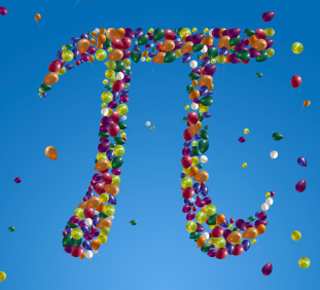GCSE Maths - 60 Enjoyable Quizzes for Fast Revision

You probably know Mathematics as ‘Maths’ or possibly, ‘that subject that gives me a headache’.

We’ve all been there. When a formula or equation just isn’t making sense, it can feel like your brain is full of custard. Luckily, we’re on hand to help with GCSE Maths revision. We’ve got enough angles, percentages, ratios, statistics and vectors to satisfy even the most enquiring minds.

We’ll start with the basics. All our GCSE Maths quizzes are labelled either ‘(F)’ or ‘(H)’. It probably doesn’t take a cryptographer to work out they stand for Foundation and Higher. Everyone should complete the Foundation quizzes and at least have a stab at the Higher ones. If you know you’re sitting the Higher paper, aim to complete all those too!

You’ve got a lot of stuff to learn in GCSE Maths and we’re here to help. We know Maths textbooks can be dreary (and we also know you really want to throw them in the sea) so what we’ve done is compile all the key stuff from the National Curriculum and put it into engaging and enjoyable Maths quizzes. They’re quick, in depth and cover important things you need to learn.

You can complete our Maths quizzes anywhere you fancy - on the sofa, in the car, on the Moon or maybe on the bus. We make our quizzes portable so you don’t have to be stuck at a desk when you don’t want to be.

When you master our GCSE Maths quizzes, you’ll feel like a huge pile of angles and sequences have been lifted from your shoulders. You can get that ‘go get em’ attitude back and walk into your exams feeling confident that you’ve taken the time to revise. Talking of revision, take a look at our Revising For GCSE Exams blog. It’s got plenty of help and advice to prepare you for the exams.

Clicking fingers at the ready, Maths Whizz Kids. It’s time to get quizzing.

Find out all about GCSEs by reading our GCSE In Detail blog.

Quiz Title Options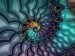Algebra - Factorising (F)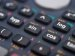Algebra 01 (F)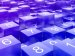Algebra 01 (H)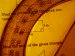Algebra 02 (F)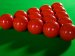Angles (F)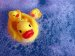Area (F)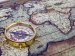Bearings (F)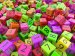BIDMAS (F)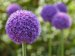Circles (F)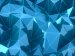Congruence (F)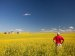Conversion Problems (Imperial to and from Metric) (F)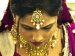Conversion Problems (Metric to Metric) (F)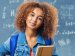Decimals (F)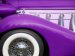Direct and Inverse Variation (H)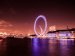Distance, Speed and Time Problems (F)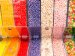Estimation in Arithmetical Calculations (F)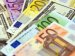Foreign Currency and Exchange Rates (F)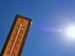Formulas (H)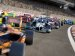Formulas and Equations (F)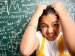Fractions (F)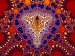HCF and Lowest Common Multiple (F)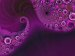Indices and Roots (F)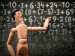Linear Equations (Numerical) (F)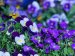Linear Equations (Word Problems) (F)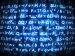Linear Inequalities (F)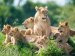Logic (F)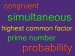Mathematical Definitions 01 (F)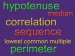Mathematical Definitions 02 (F)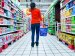Money, Profit and Loss Problems (F)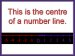Number Lines and Solution Sets (F)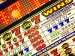Numbers (Classification) (F)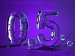Percentages (F)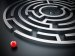Perimeter (F)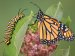Pictorial Representation of Data (F)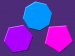Polygons (F)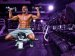Powers (H)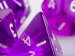Probability (F)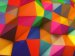Pythagoras’ Theorem (F)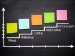Quadrants and Axes (F)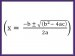Quadratic Equations (H)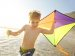Quadrilaterals (Properties) (F)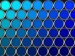Ratio and Proportion (F)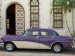Ratio, Proportion and Percentages (H)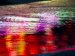Reflections, Translations and Rotations (F)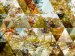Right-Angled Trigonometry (F)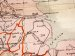Scale Drawings and Maps (F)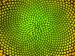Sequences (F)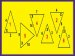Similarity (F)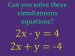Simultaneous Equations (F)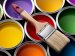Simultaneous Equations (H)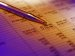Statistics (Mean, Mode, Median and Range) (F)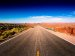Straight Line (F)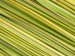Straight Line (H)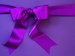Surface Area (F)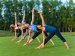Triangles (F)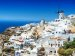Unitary Method of Solution (F)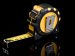Upper & Lower Bounds (H)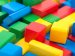Volume (F)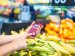Whole Number Division (F)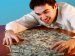Writing Numbers including Standard Form (F)

### GCSE Maths Revision - Not Easy but it's Fun!

We can't pretend to be able to make it a piece of cake to solve complex mathematical problems but at least we will try and make it fun!

We use cookies to make your experience of our website better.

To comply with the new e-Privacy directive, we need to ask for your consent -

help desk software